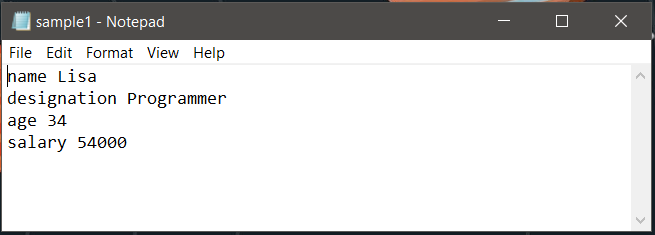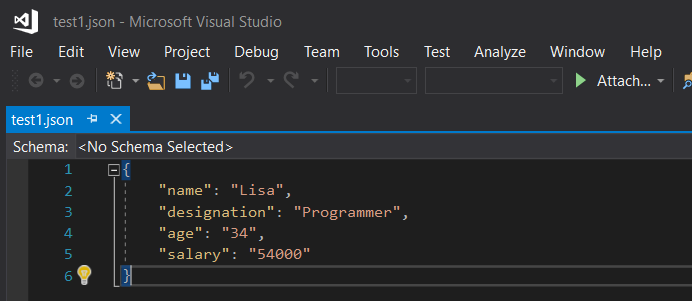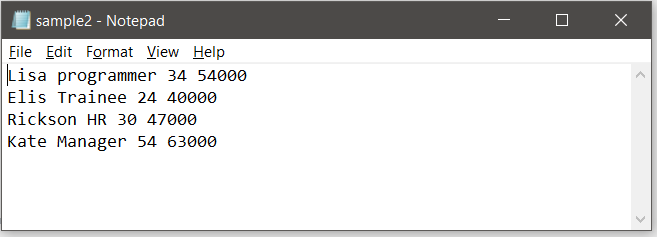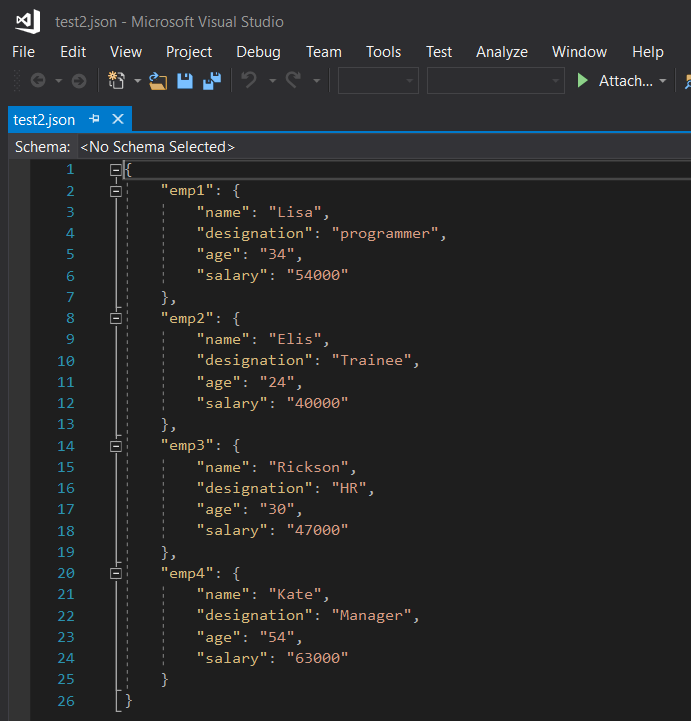# Convert Text file to JSON in Python

## Convert Text file to JSON in Python

In Python, converting a text file to JSON (JavaScript Object Notation) format is a common task for transmitting data between web applications and servers. Luckily, the process can be made easy with the help of the built-in json module. This module allows Python objects to be easily converted into their corresponding JSON objects, which can then be written to a file.

In this article, we will explore the various methods of converting text files to JSON in Python. We will begin by examining the basic process of converting a text file containing a single person’s details to a JSON file. Then, we will move on to more complex scenarios, such as when the text file contains multiple records. We will also discuss different parameters that can be used to improve the readability of the resulting JSON file. By the end of this article, you will have a comprehensive understanding of how to convert text files to JSON format in Python.

See the following table given below to see serializing JSON i.e. the process of encoding JSON.

Python object JSON object
dict object
list, tuple array
str string
int, long, float numbers
True true
False false
None null

To handle the data flow in a file, the JSON library in Python uses `dump()` function to convert the Python objects into their respective JSON object, so it makes easy to write data to files.

Syntax:

json.dump()

Various parameters can be passed to this method. They help in improving the readability of the JSON file. They are :

• dict object : the dictionary which holds the key-value pairs.
• indent : the indentation suitable for readability(a numerical value).
• separator : How the objects must be separated from each other, how a value must be seperated from its key. The symbols like “, “, “:”, “;”, “.” are used
• sort_keys : If set to true, then the keys are sorted in ascending order

Here the idea is to store the contents of the text as key-value pairs in the dictionary and then dump it into a JSON file. A simple example is explained below. The text file contains a single person’s details. The text1.txt file looks like:Now to convert this to JSON file the code below can be used:

 `# Python program to convert text` `# file to JSON` ` ` ` ` `import` `json` ` ` ` ` `# the file to be converted to ` `# json format` `filename ``=` `'data.txt'` ` ` `# dictionary where the lines from` `# text will be stored` `dict1 ``=` `{}` ` ` `# creating dictionary` `with ``open``(filename) as fh:` ` ` `    ``for` `line ``in` `fh:` ` ` `        ``# reads each line and trims of extra the spaces ` `        ``# and gives only the valid words` `        ``command, description ``=` `line.strip().split(``None``, ``1``)` ` ` `        ``dict1[command] ``=` `description.strip()` ` ` `# creating json file` `# the JSON file is named as test1` `out_file ``=` `open``(``"test1.json"``, ``"w"``)` `json.dump(dict1, out_file, indent ``=` `4``, sort_keys ``=` `False``)` `out_file.close()`

When the above code is executed, if a JSON file exists in the given name it is written to it, otherwise, a new file is created in the destination path and the contents are written to it.

Output:Note the below line of code:

`command, description = line.strip().split(None, 1)`

Here split(None, 1) is used to trim off all excess spaces between a key-value pair and ‘1’ denotes split only once in a line. This ensures in a key-value pair, the spaces in the value are not removed and those words are not split. Only the key is separated from its value.

How to convert if multiple records are stored in the text file ?
Let us consider the following text file which is an employee record containing 4 rows.The idea is to convert each employee’s detail into an intermediate dictionary and append that to one main resultant dictionary. For each intermediate dictionary a unique id is created and that serves as the key. Thus here the employee id and an intermediate dictionary make a key-value pair for the resultant dictionary to be dumped.

 `# Python program to convert text` `# file to JSON` ` ` ` ` `import` `json` ` ` ` ` `# the file to be converted` `filename ``=` `'data.txt'` ` ` `# resultant dictionary` `dict1 ``=` `{}` ` ` `# fields in the sample file ` `fields ``=``[``'name'``, ``'designation'``, ``'age'``, ``'salary'``]` ` ` `with ``open``(filename) as fh:` `     ` ` ` `     ` `    ``# count variable for employee id creation` `    ``l ``=` `1` `     ` `    ``for` `line ``in` `fh:` `         ` `        ``# reading line by line from the text file` `        ``description ``=` `list``( line.strip().split(``None``, ``4``))` `         ` `        ``# for output see below` `        ``print``(description) ` `         ` `        ``# for automatic creation of id for each employee` `        ``sno ``=``'emp'``+``str``(l)` `     ` `        ``# loop variable` `        ``i ``=` `0` `        ``# intermediate dictionary` `        ``dict2 ``=` `{}` `        ``while` `i<``len``(fields):` `             ` `                ``# creating dictionary for each employee` `                ``dict2[fields[i]]``=` `description[i]` `                ``i ``=` `i ``+` `1` `                 ` `        ``# appending the record of each employee to` `        ``# the main dictionary` `        ``dict1[sno]``=` `dict2` `        ``l ``=` `l ``+` `1` ` ` ` ` `# creating json file        ` `out_file ``=` `open``(``"test2.json"``, ``"w"``)` `json.dump(dict1, out_file, indent ``=` `4``)` `out_file.close()`

The attributes associated with each column is stored in a separate list called ‘fields’. In the above code, Each line is split on the basis of space and converted into a dictionary. Each time the line print(attributes) get executed, it appears as given below.

```['Lisa', 'programmer', '34', '54000']
['Elis', 'Trainee', '24', '40000']
['Rickson', 'HR', '30', '47000']
['Kate', 'Manager', '54', '63000']
```

The JSON file created by this code looks like :## Convert nested text file to nested JSON:

If you have a nested text file that you want to convert to nested JSON, you can use the `json` module and some string manipulation functions in Python. Here’s an example code snippet:

```import json # the text file to be converted filename = 'data.txt' (adsbygoogle = window.adsbygoogle || []).push({}); # read the text file and split it into sections with open(filename, 'r') as textfile: sections = textfile.read().split('\n\n') # convert each section to a dictionary and store it in a list records = [] for section in sections: # split the section into lines and remove extra whitespace lines = [line.strip() for line in section.split('\n')] # create a dictionary from the lines record = {} for line in lines: key, value = line.split(':') record[key.strip()] = value.strip() records.append(record) # convert the list of dictionaries to a nested JSON object jsonobj = json.dumps(records, indent=4) (adsbygoogle = window.adsbygoogle || []).push({}); # write the JSON object to a file with open('data.json', 'w') as jsonfile: jsonfile.write(jsonobj) ```

In this example, the text file contains sections separated by two newline characters (`\n\n`). Each section represents a record with key-value pairs separated by a colon (`:`). The code reads the text file, splits it into sections, converts each section to a dictionary, and stores the dictionaries in a list. Finally, the list of dictionaries is converted to a nested JSON object and written to a file.

Last Updated on April 16, 2023 by admin

## Python – Get all substrings of given stringPython – Get all substrings of given string

Python | Get all substrings of given string There are many problems in which we

## Creating a Pandas dataframe column based on a given conditionCreating a Pandas dataframe column based on a given condition

Creating a Pandas dataframe column based on a given condition   While operating on data,

## How to get column names in Pandas dataframeHow to get column names in Pandas dataframe

While analyzing the real datasets which are often very huge in size, we might need

## Passing function as an argument in PythonPassing function as an argument in Python

Passing function as an argument in Python A function can take multiple arguments, these arguments

## Create empty dataframe in PandasCreate empty dataframe in Pandas

The Pandas Dataframe is a structure that has data in the 2D format and labels

## Python – Pandas DataFrame.transposePython – Pandas DataFrame.transpose

Pandas DataFrame.transpose Pandas DataFrame.transpose() function transpose index and columns of the dataframe. It reflect the

## Python Dictionary has_key()Python Dictionary has_key()

Python | Dictionary has_key() Dictionary in Python is an unordered collection of data values, used to

## Access Modifiers in Python : Public, Private and ProtectedAccess Modifiers in Python : Public, Private and Protected

Access Modifiers in Python : Public, Private and Protected Various object-oriented languages like C++, Java,